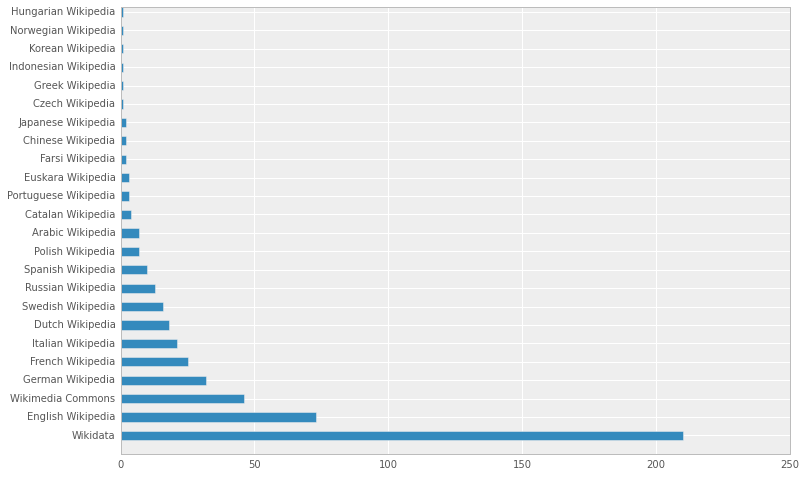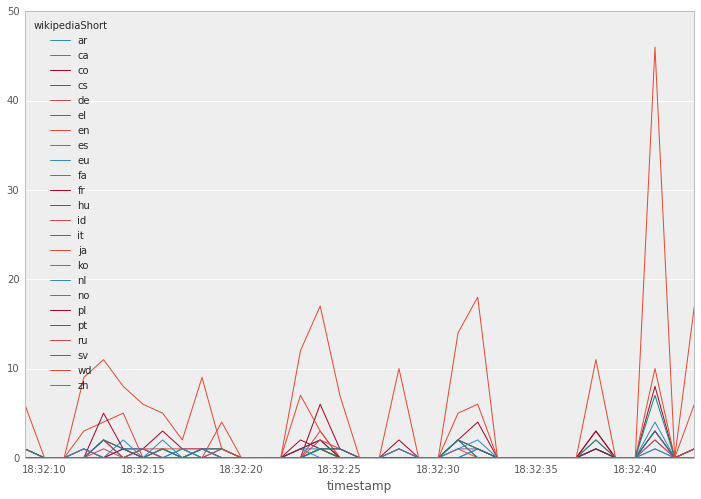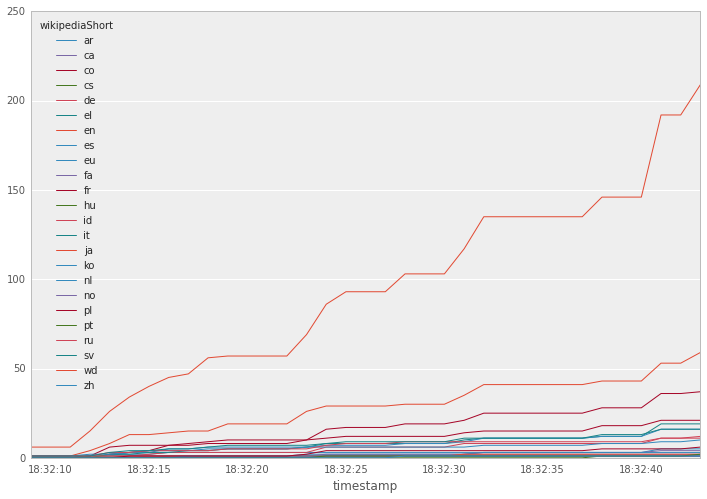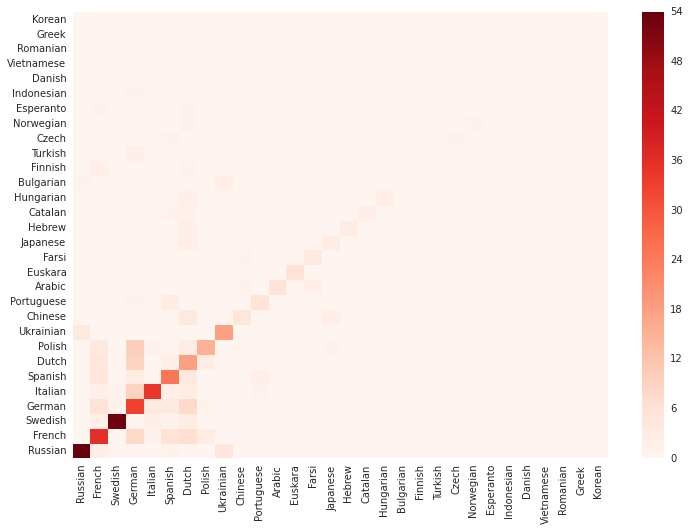# Wikipedia edit stream demo¶

A demo of Snake Charmer, originally presented at a PyData London meetup in August 2014, showing:

• Reading data from a WebSockets stream in real time, in a background thread
• Pulling this data into Pandas for analysis and visualization
• Training a Vowpal Wabbit language classifier on this stream, in real time
• Visualizing the classifier's behaviour using Matplotlib and D3

The demo is designed to be run using Snake Charmer release a18945d or later.

Wikipedia streaming is powered by the wikipedia_updates function, which is based on the stream.py script provided with edsu/wikistream.

In :
import re
import json
import time

from collections import defaultdict
from itertools import chain
from requests import post, get
from math import sqrt

from wabbit_wappa import VW

import numpy as np

import pandas as pd
pd.options.display.mpl_style = 'default'

import seaborn as sb
import matplotlib.pylab as pylab
import matplotlib.pyplot as plt
pylab.rcParams['figure.figsize'] = (12.0, 8.0)
pylab.rcParams['font.family'] = 'Bitstream Vera Sans'


This function talks to the wikistream server, which provides push notifications of Wikipedia edits via Socket.io.

Call it with a callback function of your own devising, that takes a single argument. Every time a Wikipedia edit occurs, your callback will be called, with a dict containing the details of the edit, e.g.:

{'flag': '',
'namespace': 'article',
'userUrl': 'http://en.wikipedia.org/wiki/User:137.44.83.167',
'url': 'http://en.wikipedia.org/w/index.php?diff=619400522&oldid=618259633',
'wikipediaLong': 'English Wikipedia',
'wikipediaShort': 'en',
'user': '137.44.83.167',
'comment': '',
'newPage': False,
'pageUrl': 'http://en.wikipedia.org/wiki/Battle_of_Trafalgar',
'unpatrolled': False,
'robot': False,
'anonymous': True,
'delta': 2,
'channel': '#en.wikipedia',
'wikipediaUrl': 'http://en.wikipedia.org',
'wikipedia': 'English Wikipedia',
'page': 'Battle of Trafalgar'}

Your callback should return True if it wants to keep receiving edits, or False to stop.

In :
def wikipedia_updates(callback):
endpoint = "http://wikistream.inkdroid.org/socket.io/1"
session_id = post(endpoint).content.decode('utf-8').split(':')
xhr_endpoint = "/".join((endpoint, "xhr-polling", session_id))

while True:
t = time.time() * 1000000
response = get(xhr_endpoint, params={'t': t}).content.decode('utf-8')

chunks = re.split(u'\ufffd[0-9]+\ufffd', response)
for chunk in chunks:
parts = chunk.split(':', 3)
if len(parts) == 4:
try:
except:
raise ValueError('Received non-json data: ' + chunk)
return


## Watching stream activity in real time¶

This is a callback function that collects the edits into a list called edits, for offline analysis later. It runs until max_edits edits have been received.

A timestamp field is added to each edit, as they don't have this by default.

Run this cell to start gathering the data in the background.

### Note¶

If at any point you get an error message about non-json data, wait a few seconds, then restart the IPython kernel and retry from the first cell. This seems to be an intermittent problem with the server -- or possibly the requests library?

In :
max_edits = 500
edits = []

def simple_callback(edit):
edit['timestamp'] = time.time()
edits.append(edit)
return len(edits) < max_edits

thr.start()


We can watch the data as it arrives. Run the following cell repeatedly, until it tells you it has finished.

In :
reg = re.compile(r'^.*wiki/(.*)')

c = len(edits)
n = min(5, c)
print('Last %d of %d edits...\n' % (n, c))
for edit in edits[-n:]:
wiki_name = edit['wikipediaLong']
page_name = reg.match(edit['pageUrl']).group(1)
comment = edit['comment']
print('\t'.join((wiki_name, page_name, comment)), '\n', flush=True)
if c == max_edits:
print('Done.\n')
else:
print('Still streaming.\n')

Last 5 of 500 edits...

Wikidata	Q1022537	/* wbsetdescription-add:1|nn */ Auto-description for Norwegian (Nynorsk)

Wikidata	Q1029016	/* wbsetdescription-add:1|nb */ Auto-description for Norwegian (Bokmål)

Wikidata	Q17744235	/* wbeditentity-create:0| */

English Wikipedia	Wikipedia:Tutorial/Editing/sandbox

Wikidata	Q2068332	/* wbeditentity-update:0| */ Added: [[eu:Rhynchobatus luebberti]]

Done.



## Import into pandas¶

Let's add all these edits into a DataFrame to make them easier to work with.

In :
df = pd.DataFrame(edits)
df['timestamp'] = pd.to_datetime(df['timestamp'], unit='s')

Out:
anonymous channel comment delta flag namespace newPage page pageUrl robot timestamp unpatrolled url user userUrl wikipedia wikipediaLong wikipediaShort wikipediaUrl
0 False #en.wikipedia /* top */ -5 M article False Android version history http://en.wikipedia.org/wiki/Android_version_h... False 2014-08-31 18:32:09.978374 False http://en.wikipedia.org/w/index.php?diff=62360... JordanKyser22 http://en.wikipedia.org/wiki/User:JordanKyser22 English Wikipedia English Wikipedia en http://en.wikipedia.org
1 False #ru.wikipedia /* Противники */ 12 article False Чёрно-жёлто-белый флаг http://ru.wikipedia.org/wiki/Чёрно-жёлто-белый... False 2014-08-31 18:32:09.978403 False http://ru.wikipedia.org/w/index.php?diff=65199... Камарад Че http://ru.wikipedia.org/wiki/User:Камарад Че Russian Wikipedia Russian Wikipedia ru http://ru.wikipedia.org
2 False #wikidata.wikipedia /* wbcreateclaim-create:1| */ [[Property:P1459... 346 B article False Q17744225 http://wikidata.org/wiki/Q17744225 True 2014-08-31 18:32:09.978426 False http://www.wikidata.org/w/index.php?diff=15466... Reinheitsgebot http://wikidata.org/wiki/User:Reinheitsgebot Wikidata Wikidata wd http://wikidata.org
3 False #nl.wikipedia Linkonderhoud -232 article False Beersel http://nl.wikipedia.org/wiki/Beersel False 2014-08-31 18:32:09.978446 False http://nl.wikipedia.org/w/index.php?diff=41990... Smile4ever http://nl.wikipedia.org/wiki/User:Smile4ever Dutch Wikipedia Dutch Wikipedia nl http://nl.wikipedia.org
4 False #wikidata.wikipedia /* wbcreateclaim-create:1| */ [[Property:P1412... 405 article False Q3035478 http://wikidata.org/wiki/Q3035478 False 2014-08-31 18:32:09.978467 False http://www.wikidata.org/w/index.php?diff=15466... Jura1 http://wikidata.org/wiki/User:Jura1 Wikidata Wikidata wd http://wikidata.org

## Visualizing the total number of edits in each wiki¶

This is a one-liner in pandas!

In :
p = df.wikipedia.value_counts().plot(kind='barh')## Checking and filtering bot activity¶

Wikidata is very busy -- is it all bots?

In :
df[df.wikipediaShort=='wd'].robot.value_counts()

Out:
True     188
False     22
dtype: int64

## Time series analysis¶

To make time series analysis easier, let's turn the raw edits into counts per second.

We can use a bit of a trick here. Pivot the dataframe, keeping the timestamp as the index, making the wiki short names the new columns, and the value of the delta field for the cells. What is delta? That doesn't actually matter! We'll see why, shortly.

### Usage note¶

The following cell originally used

pivot(index='timestamp',
columns='wikipediaShort',
values='delta')

but this doesn't allow duplicates in the field to be used as the index. So if you're unlucky enough to get two edits in the same microsecond, it'll fail.

pivot_table, however, handles these properly.

In :
ts = pd.pivot_table(df, values='delta', index='timestamp', columns='wikipediaShort')

Out:
wikipediaShort ar ca co cs de el en es eu fa ... ja ko nl no pl pt ru sv wd zh
timestamp
2014-08-31 18:32:09.978374 NaN NaN NaN NaN NaN NaN -5 NaN NaN NaN ... NaN NaN NaN NaN NaN NaN NaN NaN NaN NaN
2014-08-31 18:32:09.978403 NaN NaN NaN NaN NaN NaN NaN NaN NaN NaN ... NaN NaN NaN NaN NaN NaN 12 NaN NaN NaN
2014-08-31 18:32:09.978426 NaN NaN NaN NaN NaN NaN NaN NaN NaN NaN ... NaN NaN NaN NaN NaN NaN NaN NaN 346 NaN
2014-08-31 18:32:09.978446 NaN NaN NaN NaN NaN NaN NaN NaN NaN NaN ... NaN NaN -232 NaN NaN NaN NaN NaN NaN NaN
2014-08-31 18:32:09.978467 NaN NaN NaN NaN NaN NaN NaN NaN NaN NaN ... NaN NaN NaN NaN NaN NaN NaN NaN 405 NaN

5 rows × 24 columns

Then, by resampling the time series to second resolution, and applying the count aggregate, we get edits-per-second.

We used the delta field as the value to be placed into each cell, not because we care about delta, but because the resample method only works on numerics.

In :
sec_counts = ts.resample('s', how='count')

Out:
wikipediaShort ar ca co cs de el en es eu fa ... ja ko nl no pl pt ru sv wd zh
timestamp
2014-08-31 18:32:09 0 0 0 0 0 0 1 0 0 0 ... 0 0 1 0 0 0 1 1 6 0
2014-08-31 18:32:10 0 0 0 0 0 0 0 0 0 0 ... 0 0 0 0 0 0 0 0 0 0
2014-08-31 18:32:11 0 0 0 0 0 0 0 0 0 0 ... 0 0 0 0 0 0 0 0 0 0
2014-08-31 18:32:12 0 0 0 0 0 0 3 1 1 1 ... 1 0 1 0 0 0 0 0 9 0
2014-08-31 18:32:13 2 0 2 0 1 0 4 0 0 0 ... 0 0 0 0 0 0 2 2 11 0

5 rows × 24 columns

## Visualizing activity over time¶

This is easy, now we have the data in the right format.

In :
p = sec_counts.plot()Not so easy to read! (Even without taking into account the terrible default legend placement.)

What if we make it a cumulative sum instead, so we can see how activity 'mounts up' over time?

That's much nicer. Although you'd really want to select just the most active or interesting wikis and show those, because with 20+ lines, the colours become hard to tell apart. This is left as an exercise :-)

In :
p = sec_counts.cumsum().plot()## Training a model in real time with Vowpal Wabbit¶

Here's the fun bit. We're going to restart the stream, and as the data comes in, we're going to feed it to Vowpal Wabbit to incrementally train a language recognizer. Or more strictly, a wiki recognizer, as we want to teach it to distinguish e.g. French Wikipedia articles from Polish Wikipedia articles -- using only the distribution of characters, and character n-grams, in their titles.

Jargon buster: Character n-grams, sometimes called shingles, are subsequences of length n. So if n == 2, it's all the consecutive pairs of characters in the input string.

We'll use an adaptive online learning approach: only update the model when we find an example that it can't correctly identify already. VW makes this very efficient and effective.

First let's define a helper function to generate character n-grams, for n from n1 to n2. That is, if n1 == 2 and n2 == 4, then you'll get bigrams, trigrams and tetragrams (is that a word?). If n1 == 1, as in the example below, you'll get all the individual characters too. Also, if you set include_words=True, it will include all the complete words yielded by splitting the string on whitespace.

The function returns an iterator over all the resulting n-grams. Any which consist purely of space characters are dropped.

In :
# Helper function to get all length-n ngrams
def _ngrams(text, n):
return (text[i:i+n]
for i in range(len(text) - (n-1)))

# Helper function to get all words, padded by a space on either side
def _words(text):
return (s.center(len(s) + 2)
for s in text.split())

# Helper function to filter out tokens that are all whitespace or numerals
def _filter(word):
return (not word.strip().isspace()) and (not word.strip().isnumeric())

# Get all ngrams for n1...n2, and optionally whole words
def ngrams(text, n1, n2, include_words=False):
if n1 < 1:
raise ValueError('n1 must be 1 or more')
if n2 < n1:
raise ValueError('n2 must not be less than n1')
return filter(_filter,
chain(_words(text) if include_words else (),
chain.from_iterable(_ngrams(text, n)
for n in range(n1, n2+1))))

print(list(ngrams(' the quick brown fox 1997', 1, 2, include_words=True)))

[' the ', ' quick ', ' brown ', ' fox ', ' ', 't', 'h', 'e', ' ', 'q', 'u', 'i', 'c', 'k', ' ', 'b', 'r', 'o', 'w', 'n', ' ', 'f', 'o', 'x', ' ', ' t', 'th', 'he', 'e ', ' q', 'qu', 'ui', 'ic', 'ck', 'k ', ' b', 'br', 'ro', 'ow', 'wn', 'n ', ' f', 'fo', 'ox', 'x ']


The next function contains filtering logic, defining what kinds of edit we are interested in. It also performs some simple cleaning of the input data.

We'll filter out Wikidata edits (wd), as these just have IDs, as well as Commons files (co), non-article pages like user homepages, and also English Wikipedia (en) -- to keep things interesting.

The function returns just the page name if it's one we want to keep, or False if it's not. Parentheses and commas are removed, and hyphens and underscores replaced by spaces. A space is added at the start and end of the name, to help with ngram generation later.

In :
skip_reg = re.compile(r'[(),]+')
split_reg = re.compile(r'[ _-]+')

def page_name(edit):
if edit['wikipediaShort'] == 'wd' or \
edit['wikipediaShort'] == 'en' or \
edit['wikipediaShort'] == 'co' or \
edit['namespace'] != 'article':
return False
name = split_reg.sub(' ', skip_reg.sub('', edit['page'].lower().strip()))
return name.center(len(name) + 2)

test_edit = {'wikipediaShort': 'xx', 'namespace': 'article', 'page': 'Testing, Testing_ (one-two) '}
print(page_name(test_edit))

 testing testing one two


We'll use a ringbuffer to keep track of predictions and actual values over the last k tests. In this kind of online learning scenario, we don't really worry how much about how the model was performing on historical data -- we just want to know that it is performing well on current data.

Here are a few convenience functions for dealing with the ringbuffer, which is implemented as a Numpy array with k rows, and a column each for the actual and predicted values. It's initialized with -1 in every cell, so we can tell when a row has yet to be filled in.

add_entry uses a Numpy trick (np.roll) to redefine the start and end of an array, while copying as little data as possible.

In :
# Create a new ringbuffer for the given number of predictions
def new_ringbuffer(capacity):
return np.zeros((capacity, 2), dtype=np.int8) - 1

# Calculate accuracy (% correct predictions) over those rows which have been filled in
def accuracy(ringbuffer):
# Filter identifying rows which have been filled in
valid = np.sum(ringbuffer, axis=1) >= 0
# Filter identifying rows where prediction == correct value
matched = ringbuffer[:,0] == ringbuffer[:,1]
# Accuracy is number of rows which are valid AND matched, over number of valid rows
return np.sum(np.logical_and(valid, matched)) / np.sum(valid)

# Return the number of valid (filled-in) rows
def valid_size(ringbuffer):
return np.sum(np.sum(ringbuffer, axis=1) >= 0)

# Add a new test result to the ringbuffer, deleting the oldest entry if necessary,
# and returning a reference to the modified buffer
capacity = len(ringbuffer)
# Overwrite the bottom row of the array
ringbuffer[capacity-1, :] = (actual, predicted)
# Roll the newest entry around to the top of the array, pushing the rest down
return np.roll(ringbuffer, 1, axis=0)


All the action happens in the next cell.

It starts the VW server with the appropriate options. If you get a memory allocation error, reduce the value of hashbits, although this may impact accuracy.

It also defines and starts a new callback, which breaks the title of each page into n-grams, and tests them against the current model. If the model classified this edit's language correctly, we don't change it. But if it classifies incorrectly, we pass it the same edit as a training instance.

The reported accuracy might fluctuate to begin with, but then should settle, and gradually rise (not necessarily in a monotonic way) as data comes in.

This may be an over-estimate of accuracy if multiple edits to the same page appear within the last k edits. For a more conservative estimate, we should filter out duplicates. But this is just a demo...

You can set a maximum number of training instances to process, or just interrupt the kernel if you get bored of waiting.

In [ ]:
# How many classes to allow in the model (must be provided up front)
max_expected_classes = 30

# When to stop, if not interrupted
max_edits = 100000

# How many tests to calculate score over
k = 500

# Ringbuffer to hold results of each test
rb = new_ringbuffer(k)

# How many bits in range of VW hash function:
# larger = potentially better accuracy but more memory
hashbits = 29

# How many edits we've received, and trained on, respectively
trained = 0

# List of the wiki names we've seen, to be populated as we go along
labels = []

# Maps each wiki name -> its index in labels list
label_to_idx = {}

def vw_callback(edit):

name = page_name(edit)
if not name:
return True

# Set up mappings for this wiki if this is the first time we've seen it
label = edit['wikipedia'].replace(' Wikipedia', '')
if label in label_to_idx:
idx = label_to_idx[label]
else:
# If we have exhausted our preset class limit, just skip it! Bit kludgey...
if len(labels) == max_expected_classes:
print("Ignoring class %d" % len(labels))
return True
labels.append(label)
idx = len(labels) - 1
label_to_idx[label] = idx

# Generate binary features -- unigrams, bigrams, trigrams, and whole words
features = set(ngrams(name, 1, 3, include_words=True))

# Test example first -- does model get it right?
raw_line = vw.make_line(features=features)
#    print(raw_line)
result = vw.send_line(raw_line).prediction
prediction = int(result) - 1 # NB VW FEATURE LABELS START AT 1

# Train on this example only if prediction was wrong
if idx == prediction:
#print('%s [%s] OK' % (name, labels[idx]), flush=True)
pass
else:
actual = idx if idx >= len(labels) else labels[idx]
predicted = prediction if prediction >= len(labels) else labels[prediction]
tested = valid_size(rb)
print('%s [%s] Failed!\nPrediction: %s, Received: %d, Trained: %d, Last-%d Accuracy: %0.3f'
% (name, actual, predicted, received, trained, tested, accuracy(rb)),
flush=True)
raw_line = vw.make_line(idx+1, features=features)
vw.send_line(raw_line)
trained += 1

# Stop if we've hit max_edits

# Start up local VW process
vw = VW(loss_function='hinge', b=hashbits, ect=max_expected_classes, hash='all', q='nn')
print(vw.command)

try:
finally:
vw.close()


## Visualizing language similarity¶

When the model mispredicts, which wikis is it most likely to get confused?

This could give us some measure of the similarity of those languages -- in the limited domain of "Wikipedia pages" at least.

If we visualize these relationships, do they roughly match what we'd expect from a non-expert idea of language similarity? Or do they reflect noise in the model -- artefacts of VW's feature hashing process?

First do some preprocessing to get a dense confusion matrix, sorted so more popular wikis come first.

In :
confusion = np.zeros((len(labels), len(labels)))
for (x, y) in rb:
confusion[x,y] += 1
samples_per_wiki = np.asarray(confusion.sum(axis=1))
sorted_idxs = np.argsort(samples_per_wiki)[::-1]
sorted_confusion = confusion[sorted_idxs,:][:,sorted_idxs]
sorted_confusion.shape

Out:
(30, 30)

Visualize the confusion matrix in Matplotlib.

The left edge represents the pages' real languages, the bottom edge shows what the model identified them as, at least in its last k tests. So, shading along the diagonal represents correct predictions, and shading off the diagonal represents errors.

The values are absolute counts, so this shows the relative popularity of each wiki as well as the error distribution.

In :
fig, ax = plt.subplots(1)
p = ax.pcolormesh(sorted_confusion, cmap='Reds')
fig.colorbar(p)

labels_array = np.array(labels)

ax.set_yticks(np.arange(sorted_confusion.shape)+0.5, minor=False)
ax.set_xticks(np.arange(sorted_confusion.shape)+0.5, minor=False)
ax.set_xticklabels(labels_array[sorted_idxs], minor=False)
ax.set_yticklabels(labels_array[sorted_idxs], minor=False)
plt.xticks(rotation=90)
plt.show()Alternatively, we can turn it into a labelled list of edge weights, write it out to a CSV, and render it in D3.

For this visualization, let's normalize by the number of actual occurrences of each wiki in the test set, so the weights indicate the proportion of the samples for each wiki source that were mistakenly predicted for target instead. Also we'll need to set the diagonal to 0 to remove loops from the visualization.

Mouseover the nodes in the graph to see the wiki name. Drag the nodes to rearrange the graph.

In :
normalized = sorted_confusion / sorted_confusion.sum(axis=1)
np.fill_diagonal(normalized, 0)
edge_weights = pd.DataFrame(data=normalized,
index=labels_array[sorted_idxs],
columns=labels_array[sorted_idxs]).stack()
edge_weights.index.names = ('source', 'target')
edge_weights.name = 'value'